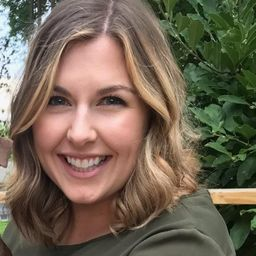Our Discord hit 10K members! 🎉 Meet students and ask top educators your questions.Join Here!Numerade Educator

# Find the volume of the solid obtained by rotating the region bounded by the given curves about the specified line. Sketch the region, the solid, and a typical disk or washer.$$y=x, y=\sqrt{x} ; \text { about } x=2$$

## $$V=\frac{8 \pi}{15}$$

#### Topics

Applications of Integration

### Discussion

You must be signed in to discuss.
##### Top Calculus 2 / BC Educators##### Catherine R.

Missouri State University##### Michael J.

Idaho State University

### Video Transcript

we're gonna find the value bounded by these graphs that I've already sketched out. And first figure that out. We want to look at the area. So the area between these equations is this part of shading and green, and we want to find volume going around X equals two. So this is X is one this is excess to and remember X equals as a vertical line. So if I'm finding the volume rotating around X equals two notice it's gonna be a washer set up because there's all this missing space as you think about it in three dimensions, you're getting kind of this doughnut bagel sort of shape as it wraps around with this big missing part in the middle. So that's a washer set up. So for the washer set up, you know, volume is pi times the integral of R squared minus R squared. And as you're thinking about your variable, you want to think about really what access you're working with An X equals two is like working with the Y axis. Notice how those are parallel. So I want to use d Y, which means my limits here within a girl are also in terms of why to the first name on the label is the Y values that bound this area. Well, the lowest Y value, I hope is obvious, is why equals zero. Because these two graphs meet at the origin. Thes graphs also meet at the 20.11 So the final y value here is one. So for my inner growing up I and then integral from 0 to 1. So then the next thing I need to figure out is what is capital R and lower case R for capital are I want to think about what would have generated a volume that had no missing pieces, a solid volume. Well, that would be if I went all the way from the edge to that axis of rotation the whole way up and down. So if I color it in like this, see how that would be a solid even as I spun it around Well, thinking about kind of like you did with area right minus left for this horizontal set up, that shaded in portion would be the vertical line to minus the curved, which is the square root of X. But remember this in and girls in terms of why So I don't want to write the square root of X here. I want to solve this to be in terms of why, in other words, make it an X equals equation. So you square both sides and realize that curved graph is really written as why squared in this case. So for capital are I'm gonna put two minus y squared. It's the same function. It's that square root equation. But I wanted to write it in terms of why so having to solve for X equals to get that written as Why squared? So for lower case, are we think about what would we have to take away? Well, this stuff I'd have to take away would go from two. Sorry, that's still little are goes from two to the straight line. The linear function is acts well again. I don't want to write this in terms of X, because my inner girls, in terms of why thankfully, here X equals wise. There's not a whole lot of to do to make that switch. I just need to write it as why. Because why was ex So this is my set up to minus y squared that quantity squared minus two minus y squared. If I were to go through and it's all this by hand, I would need to expand thes by no meals so you would want as your squaring them. You're multiplying them through your distributing all those terms. But if you have the option to calculate, this is nice one to be able type in. And this integral portion becomes 8/15 so that if I multiply for the volume, I would write eight pi over 15 and that's my final answer.Oklahoma Baptist University

#### Topics

Applications of Integration

##### Top Calculus 2 / BC Educators##### Catherine R.

Missouri State University##### Michael J.

Idaho State University